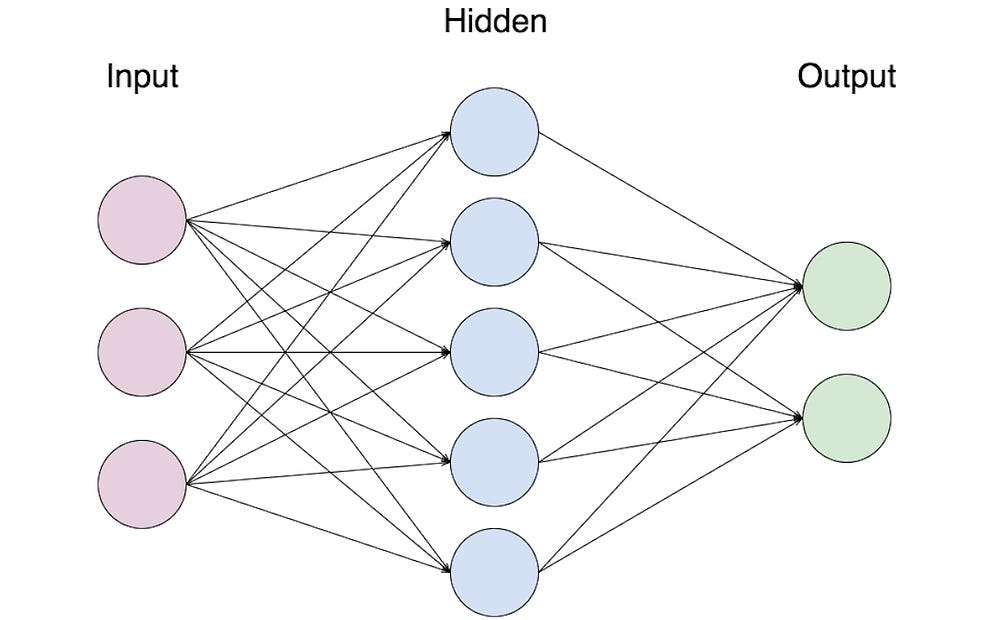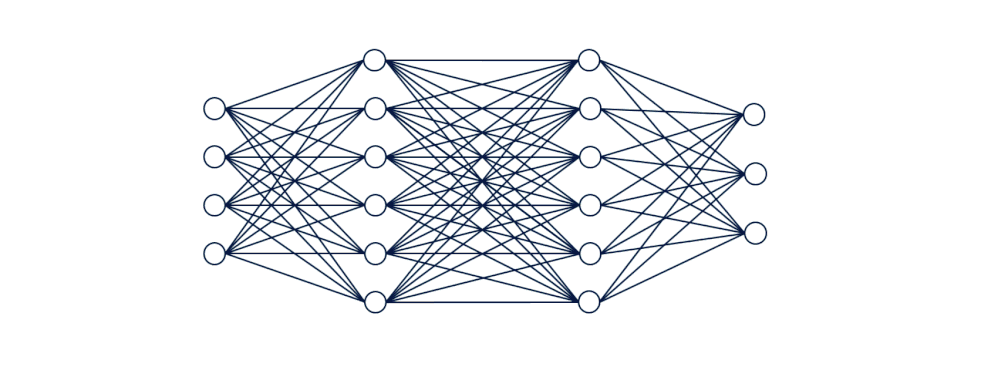# Machines with brains like ours- An intro to Deep Learning

Source: Deep Learning on Medium

What do generating photos of galaxies, creating Shakespearean style writing and improving earthquake prediction timings by 50,000% all have in common?

Surprisingly, they are all feats achieved by Artificial Intelligence (AI). Machines are becoming more intelligent and capable of learning, but the results aren’t necessarily like the Ex- Machina. In fact, the human brain itself is the inspiration for much of the technological intelligence solving problems today, through machine learning and specifically deep learning.

Machine learning is when a computer takes data and understands it, learns from it, and makes a decision but doesn’t have to be explicitly programmed to do this. Deep learning is a subfield of ML inspired by our brain’s neural networks (the structure our brain uses to process information). These neural networks are called artificial neural networks (ANNs) and sometimes interchangeably referred to as neural nets, nets, or models. They consist of a group of units called nodes or artificial neurons which (similarly to the human brain) transmit signals from one neuron to the next.A basic Artificial Neural Network

The transmission is when a neuron receives, processes and conveys signals to another neuron. This process is represented by 3 main types of layers: input, hidden and output.

### Layers in an Artificial Neural Network

Let’s take the example of a convolutional neural network, an ANN that can be used for image classification. If we want the neural net to tell the difference between a cat and a dog, it will go through these main steps.

#### Input layerThe highlighted are the input nodes which go to the first hidden layer

Each node represents an individual feature or variable from each sample data set which passes through the model. The nodes in this layer connect to each node in the next layers, the hidden layers. The connections are given weights from 0 to 1 which represent the strength of the connection. Some of the nodes in our dog vs cat input layer may be variables such as ear size, fur colour or tail length.

#### Hidden Layer

The hidden layers are any layers between the input and output. Essentially they compute a weighted sum for the connections which point to the same node in a layer. This sum is passed through an activation function, (something we will go into further detail down below), which is based on the brain and how different neurons are activated by different stimuli. The results of the activation function are passed onto the next node in the following layer and repeated over and over till the output layer.

#### Output Layer

The weights continue to change through the above process till optimized (more on this below as well). The resulting neurons in the output layer represent the values of the potential outputs. In the case of our example NN, the two nodes in the output layer would be a probability of what the net thinks it is, e.g. 0.75 dog vs 0.25 cat.

### Training the Neural Net

Training an ANN is basically solving an optimization problem. In this case, it is trying to optimize the arbitrary weights to the connections between the neuron. During training, the weight values given are constantly being updates to reach optimal value. The optimization depends on an optimization algorithm, one of which most commonly used is stochastic gradient descent (an algorithm that reduces loss).

Basically, the objective of the problem is to minimize loss function. The loss function measures the accuracy of the outcome. For example, when training a neural network to classify between cat and dog, the labelled data is provided. An image of a dog image is passed through, and then the probability (output) has come out to be 0.77 dog vs 0.23 cat. The goal is for the value for the dog to be 1.00 (to know for sure that it is a dog) and minimize the error that the results are wrong.

### How does it learn?

One pass of data is called an epoch. To learn, an ANN passes data through multiple epoch to allow the initially given random weights to develop. To improve, the loss is calculated from a given output and the gradient (the derivative of a function d(loss)/d(weight)) is multiplied by the learning rate (a number between 0.01 and 0.001).

The value from the gradient will become smaller once multiplied by the value from the learning rate. This value is taken and the old weight is replaced by the updated value.

It’s important to remember that the gradient of the cost function has a different value for each weight thus the gradient is calculated with respect to each weight. With each epoch, the weights are updated, thus getting closer and closer to the optimized value and minimizes loss. This updating of weights is essentially learning as values that need to be applied to each weight assigned based on the effect on the loss function.

### Key Takeaways

• Deep learning is a subsect of Machine learning inspired by the neural networks in our brain
• There are 3 main types of layers in an Artificial Neural Network (Input, Hidden, and Output)
• To train an ANN, the values have to be updated to minimize the cost function (how much error there is in the output)
• An ANN learns through many passes of data and iterating the weights till an optimized value

Thank you for reading! If you enjoyed this article leave a few claps and follow my Medium to keep updated with my progress in AI.